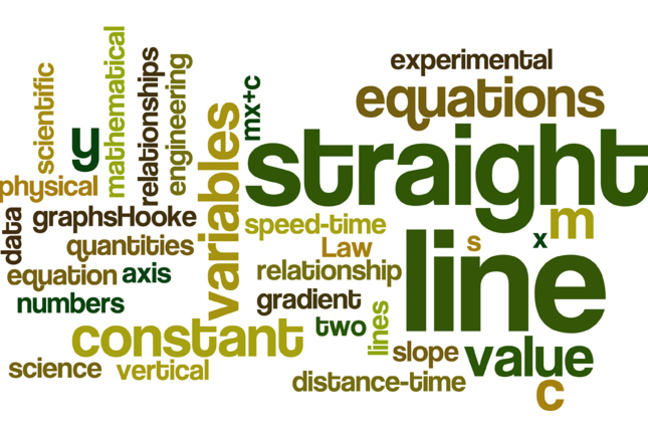## Want to keep learning?

This content is taken from the Loughborough University's online course, Getting a Grip on Mathematical Symbolism. Join the course to learn more.
3.17

## Loughborough University# Summary of Week 3

We now summarise all that we have learned:

You have spent a lot of time considering equations which have the form $$y = mx + c$$ and now know that these are mathematical representations of straight lines. You have learned about the significance of the numbers $$m$$ and $$c$$ in equations like this. Particularly, you know that the value of the constant $$m$$ is the slope or gradient of the line.

You know that the value of the constant $$c$$ tells us where the straight line crosses the vertical axis. You learned how you could fi nd the equation of a line given experimental data which suggested that a straight line relationship between two variables was present. You saw how variables other than $$x$$ and $$y$$ are used to represent physical quantities in scientifi c and engineering applications.

Finally, you saw several applications of straight line relationships such as distance-time and speed-time graphs, and Hooke’s Law.

If you have worked consistently through all the resources we have provided during the last three weeks you should be in a good position to now take the Test for this course.

Once you have a good understanding of straight line graphs and their properties you might consider reinforcing this understanding using some of the excellent on-line graphing tools available. Why not explore the excellent set of tools provided free, on-line by Desmos through which you can plot straight line graphs and more complicated curves.